# US8478005B2 - Method of performing facial recognition using genetically modified fuzzy linear discriminant analysis - Google Patents

Method of performing facial recognition using genetically modified fuzzy linear discriminant analysis Download PDF

## Info

Publication number
US8478005B2
US8478005B2 US13/084,406 US201113084406A US8478005B2 US 8478005 B2 US8478005 B2 US 8478005B2 US 201113084406 A US201113084406 A US 201113084406A US 8478005 B2 US8478005 B2 US 8478005B2
Authority
US
United States
Prior art keywords
processor
th
calculating
vectors
set
Prior art date
Legal status (The legal status is an assumption and is not a legal conclusion. Google has not performed a legal analysis and makes no representation as to the accuracy of the status listed.)
Active, expires
Application number
US13/084,406
Other versions
US20120257798A1 (en
Inventor
Amar Khoukhi
Syed Faraz Ahmed
Current Assignee (The listed assignees may be inaccurate. Google has not performed a legal analysis and makes no representation or warranty as to the accuracy of the list.)
King Fahd University of Petroleum and Minerals
Original Assignee
King Fahd University of Petroleum and Minerals
Priority date (The priority date is an assumption and is not a legal conclusion. Google has not performed a legal analysis and makes no representation as to the accuracy of the date listed.)
Filing date
Publication date
Application filed by King Fahd University of Petroleum and Minerals filed Critical King Fahd University of Petroleum and Minerals
Priority to US13/084,406 priority Critical patent/US8478005B2/en
Assigned to KING FAHD UNIVERSITY OF PETROLEUM AND MINERALS reassignment KING FAHD UNIVERSITY OF PETROLEUM AND MINERALS ASSIGNMENT OF ASSIGNORS INTEREST (SEE DOCUMENT FOR DETAILS). Assignors: AHMED, SYED FARAZ, DR., KHOUKHI, AMAR, DR.
Publication of US20120257798A1 publication Critical patent/US20120257798A1/en
Application granted granted Critical
Publication of US8478005B2 publication Critical patent/US8478005B2/en
Application status is Active legal-status Critical

• 230000001815 facial Effects 0 abstract claims description title 45
• 238000004458 analytical methods Methods 0 abstract claims description title 24
• 230000002068 genetic Effects 0 abstract claims description 34
• 238000004422 calculation algorithm Methods 0 abstract claims description 30
• 239000011159 matrix materials Substances 0 claims description 64
• 230000015654 memory Effects 0 claims description 8
• 239000003607 modifier Substances 0 claims description 8
• 230000001131 transforming Effects 0 claims description 7
• 230000001276 controlling effects Effects 0 claims description 5
• 238000009740 moulding (composite fabrication) Methods 0 claims description 5
• 239000002609 media Substances 0 claims description 4
• 238000006011 modification Methods 0 abstract description 4
• 230000004048 modification Effects 0 abstract description 4
• 238000004364 calculation methods Methods 0 abstract description 2
• 238000003860 storage Methods 0 claims 2
• 238000000513 principal component analysis Methods 0 description 16
• 230000000694 effects Effects 0 description 6
• 238000000034 methods Methods 0 description 6
• 230000014509 gene expression Effects 0 description 3
• 230000000875 corresponding Effects 0 description 2
• 239000011521 glass Substances 0 description 2
• 230000001976 improved Effects 0 description 2
• 230000003287 optical Effects 0 description 2
• 238000004891 communication Methods 0 description 1
• 238000007906 compression Methods 0 description 1
• 238000000605 extraction Methods 0 description 1
• 230000004438 eyesight Effects 0 description 1
• 239000000727 fractions Substances 0 description 1
• 230000001965 increased Effects 0 description 1
• 230000013016 learning Effects 0 description 1
• 238000005457 optimization Methods 0 description 1
• 238000007781 pre-processing Methods 0 description 1
• 230000002829 reduced Effects 0 description 1
• 230000001603 reducing Effects 0 description 1
• 239000004065 semiconductor Substances 0 description 1
• 238000000638 solvent extraction Methods 0 description 1

## Images

•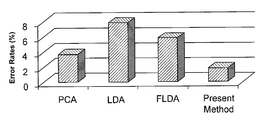•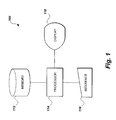•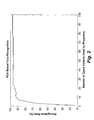•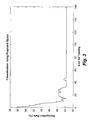•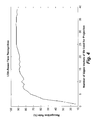•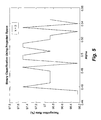•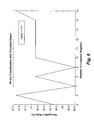•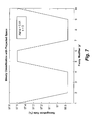•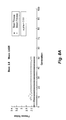•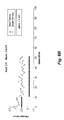•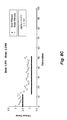•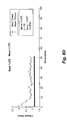•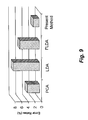•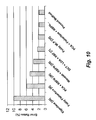## Classifications

• GPHYSICS
• G06COMPUTING; CALCULATING; COUNTING
• G06KRECOGNITION OF DATA; PRESENTATION OF DATA; RECORD CARRIERS; HANDLING RECORD CARRIERS
• G06K9/00Methods or arrangements for reading or recognising printed or written characters or for recognising patterns, e.g. fingerprints
• G06K9/62Methods or arrangements for recognition using electronic means
• G06K9/6217Design or setup of recognition systems and techniques; Extraction of features in feature space; Clustering techniques; Blind source separation
• G06K9/6232Extracting features by transforming the feature space, e.g. multidimensional scaling; Mappings, e.g. subspace methods
• G06K9/6234Extracting features by transforming the feature space, e.g. multidimensional scaling; Mappings, e.g. subspace methods based on a discrimination criterion, e.g. discriminant analysis
• GPHYSICS
• G06COMPUTING; CALCULATING; COUNTING
• G06KRECOGNITION OF DATA; PRESENTATION OF DATA; RECORD CARRIERS; HANDLING RECORD CARRIERS
• G06K9/00Methods or arrangements for reading or recognising printed or written characters or for recognising patterns, e.g. fingerprints
• G06K9/00221Acquiring or recognising human faces, facial parts, facial sketches, facial expressions
• G06K9/00288Classification, e.g. identification

## Abstract

The method of performing facial recognition using genetic algorithm-modified fuzzy linear discriminant analysis (LDA) is based on the Fisherface LDA, with a modification being made in calculation of the membership function. Particularly, the membership function is computed using a pair of parameters α and β, which are optimized by a genetic algorithm in order to minimize the recognition error.

## Description

BACKGROUND OF THE INVENTION

1. Field of the Invention

The present invention relates generally to biometrics and computer applications for facial recognition, and particularly to a method of performing facial recognition using genetically modified fuzzy linear discriminant analysis, including modification of the Fuzzy Fisherface classification scheme.

2. Description of the Related Art

Facial recognition has recently found many applications and has attracted substantial research efforts from the areas of computer vision, bio-informatics, and machine learning. The techniques used for facial recognition are broadly classified as either appearance-based or geometrical feature-based. The appearance-based techniques use the holistic features of the face image, whereas the geometrical features of the image are utilized in the latter. Some researchers have also adopted a hybrid methodology by applying the appearance-based techniques on the localized regions of the facial image.

Principal Component Analysis (PCA) is one of the most successful techniques used in face image recognition. PCA can be used to perform prediction, redundancy removal, feature extraction, and data compression, etc. PCA essentially reduces the large dimensionality of the data space. The projection of the data is in the direction of the maximum variance of the data used to find the features. However, this subspace is not necessarily optimal in terms of face classification. Large 1-D vectors of pixels are constructed from 2-D facial images, by concatenating the columns and are then projected onto the eigenvectors of the covariance matrix of the training image vectors. If there are N (the number of images) vectors of size M (rows by columns of an image), then the mean vector of all of the images is given by:

$m = 1 N ⁢ ∑ i = 1 N ⁢ x i . ( 1 )$

In PCA, the image vectors are first mean-centered. The set of T orthonormal vectors wi's is sought, forming the projection matrix W of order (M×T), and the feature vectors are then given by the following linear transformation:
y k =W T x k  (2)

PCA relies on maximizing the total scatter of the training vectors. The total scatter matrix ST is given by:

$S T = ∑ k = 1 N ⁢ ( x k - m ) ⁢ ( x k - m ) T . ( 3 )$
The scatter of the transformed feature vectors is given by WTSTW. The projection matrix WPCA satisfies the following:
W PCA=argW max |W T S T W|.  (4)

It can be shown from linear algebra that the wi are the eigenvectors of the covariance matrix C=PTP, where P is a matrix composed of the mean centered images mi as the column vectors placed side by side. Since N image vectors are summed up, the rank of the covariance matrix cannot exceed (N−1), since the vectors are mean subtracted.

The non-zero eigenvalues of the covariance matrix have corresponding orthonormal eigenvectors. The eigenvector associated with the largest eigenvalue is one that reflects the greatest variance in the image. The eigenvalues decrease very rapidly: Roughly 90% of the total variance is contained in the first 5% to 10% of the dimensions, as shown in FIG. 2.

FIG. 2 shows only the first one hundred eigenvectors used for projection, but it is clear that projection using only the first fifteen eigenvectors of the covariance matrix results in above 90% recognition accuracy. Thus, image vectors are projected onto a subspace formed by the most significant eigenvectors (i.e., the principal components) of the covariance matrix. When a test image is projected onto the N-dimensional subspace, it is classified as the class of the vector that minimizes the Euclidean distance with it.

Linear Discriminant Analysis (LDA) looks for the projection matrix that provides the best discrimination among the different classes. LDA tries to achieve this by finding a subspace in which the projected vectors of the different classes are maximally separated. The between-class scatter matrix SB and the within-class scatter matrix SW are defined as:

$S B = ∑ i = 1 c ⁢ M i ⁡ ( x i - m ) · ( x i - m ) T ( 5 ) S W = ∑ i = 1 c ⁢ ∑ x k ∈ X i ⁢ M i ⁡ ( x i - m i ) · ( x i - m ) T , ( 6 )$
where Mi is the number of training vectors in the i-th class, c is the number of distinct classes, mi is the mean of all the vectors belonging to the i-th class, and Xi represents the set of samples belonging to the i-th class, where xk is the k-th image of that class.

SW represents the scatter of the features around the mean of each class, and SB represents the scatter of features around the overall mean for all the classes. In Fisher's LDA, the aim is to maximize SB while minimizing SW, which translates to maximize the ratio between their determinants

$det ⁢  S B  det ⁢  S W  :$

$W LDA = arg ⁢ ⁢ max W ⁢  W T ⁢ S B ⁢ W   W T ⁢ S W ⁢ W  . ( 7 )$

This ratio is maximized when the column vectors of the projection matrix WLDA are the eigenvectors of SW −1SB. In order to avoid SW from becoming singular, PCA is used as a preprocessing step. Thus, the final transformation is given by the following matrix:
W T =W LDA T ·W PCA T.  (8)
LDA produces well-separated classes in a low-dimensional subspace, even under severe variations in lighting and facial expressions.

In the Fuzzy Fisherface LDA (FLDA), the basic LDA is modified. The modification is the introduction of fuzziness into the “belong-ness” of every projected vector to the classes. In the conventional approach, every vector is assumed to have a crisp membership in the class to which it belongs. However, this does not take into account the resemblance of images belonging to different classes, which occurs under varying conditions. In FLDA, a vector is assigned the membership grades for every class based upon the class label of its k nearest neighbors. This fuzzy k-nearest neighbor algorithm is used to calculate the membership grades of all the vectors. In this manner, the inter-class image resemblance is accounted for. The fuzzy C-class partitioning of the vectors defines the degrees of membership of each vector to all the classes.

In the following, μij represents the membership grade of the j-th vector in the i-th class. The membership functions satisfy the two obvious conditions:

$∑ i = 1 C ⁢ μ ij = 1 ( 9 ) 0 < ∑ j = 1 N ⁢ μ ij < N . ( 10 )$

During the training phase, the class labels of the k vectors located in the closest neighborhood of each vector is collected. Then, the membership grade of the j-th vector to i-th class is calculated using the expression as:

$μ ij = { 0.51 + 0.49 ⁢ ( n ij k ) if ⁢ ⁢ i ⁢ ⁢ is ⁢ ⁢ the ⁢ ⁢ same ⁢ ⁢ as ⁢ ⁢ the ⁢ ⁢ label ⁢ ⁢ of ⁢ ⁢ the ⁢ ⁢ j ⁢ - ⁢ th ⁢ ⁢ pattern 0.49 ⁢ ( n ij k ) ⁢ ⁢ otherwise ( 11 )$
where nij stands for the number of the neighbors of the j-th vector that belong to the i-th class. The membership allocation formula refines the membership grades of the labeled vectors, and the dominant membership has not been affected. These modified membership grades are used in the computations of the statistical properties of the patterns, such as the mean value and scatter covariance matrices SB and SW:

$m _ l = ∑ j = 1 N ⁢ μ ij p ⁢ x j ∑ j = 1 N ⁢ μ ij p ( 12 ) FS B = ∑ i = 1 C ⁢ ∑ j = 1 N ⁢ μ ij p ⁡ ( m _ l - m ) · ( m _ l - m ) T ( 13 ) FS W = ∑ i = 1 C ⁢ ∑ x k ∈ X i ⁢ μ ij p ⁡ ( x i - m _ l ) · ( x i - m _ l ) T ( 14 )$
where i=1, 2, . . . , c and p, a fuzzy modifier, is a constant that controls the influence of the fuzzy membership degree.

Although the Fuzzy Fisherface LDA modification results in improved facial recognition, there is still a need for further improvement in computer applications for facial recognition. Thus, a method of performing facial recognition using genetically modified fuzzy linear discriminant analysis solving the aforementioned problems is desired.

SUMMARY OF THE INVENTION

In conventional facial recognition methods, the relationship of each face to a particular class is assumed to be crisp. The Fuzzy Fisherface method introduces a gradual level of assignment of each face pattern to a class, using a membership grading based upon the K-Nearest Neighbor (KNN) algorithm. In the present method, the Fuzzy Fisherface Linear Discriminant Analysis method is modified by incorporating the membership grade of each face pattern into the calculation of the between-class and with-in class scatter matrices in a process referred to herein as “Complete Fuzzy LDA” (CFLDA).

Both the Fuzzy Fisherface and CFLDA methods utilize the Fuzzy-KNN algorithm. The present method further improves the assignment of class membership by improving the parameters of the membership functions. A genetic algorithm is used to optimize these parameters by searching the parameter space. Further, the genetic algorithm is used to find the optimal number of nearest neighbors to be considered during the training phase.

The present method of performing facial recognition using a genetic algorithm-modified fuzzy linear discriminant analysis can be summarized by the following set of steps: (a) establishing a set of N test face images, where N is a non-zero, positive integer; (b) establishing a set of N training face images; (c) calculating a mean vector of all test face images as

$m ′ = 1 N ⁢ ∑ i = 1 N ⁢ x i ′ ,$
where x′i represents an i-th test face image vector having a size M′, where i is an integer; (d) calculating a mean vector of all training face images as

$m = 1 N ⁢ ∑ i = 1 N ⁢ x i ,$
where xi represents an i-th training face image vector having a size M; and (e) calculating a total scatter matrix ST as

$S T = ∑ k = 1 N ⁢ ( x k - m ) ⁢ ( x k - m ) T ,$
where k is an integer.

The method proceeds further by: (f) calculating a set of orthonormal training image vectors wi, wherein the set of orthonormal vectors wi are eigenvectors of a covariance matrix C=PTP, where P is a matrix composed of mean centered images mi as column vectors, placed side by side; (g) forming a projection matrix W of order (M×T) from the set of orthonormal training image vectors wi, where T represents the total number of orthonormal training image vectors wi; (h) calculating a first projection matrix WPCA as WPCA=argW max|WTSTW|; and (i) calculating a membership grade of a j-th training image vector in the i-th class μij as

$μ ij = α + β ⁢ ⁢ ( n ij k )$
if i is the same as a label of the j-th pattern, and as

$μ ij = β ⁢ ⁢ ( n ij k )$
if i is not the same as the label of the j-th pattern, where nij represents a number of neighbors of the j-th vector that belong to the i-th class, k is an integer representing a number of nearest neighbors of the j-th training image vector, and wherein β=1−α, and α represents an offset in membership grading assigned to a training vector in its class.

The method continues by: (j) optimizing a using a genetic algorithm in order to minimize recognition error; (k) calculating a mean of all training vectors belonging to the l-th class as

$m _ l = ∑ j = 1 N ⁢ μ ij p ⁢ x j ∑ j = 1 N ⁢ μ ij p ,$
where l is an integer; (l) calculating a between-class scatter matrix SB and a within-class scatter matrix SW as

$S B = ∑ i = 1 C ⁢ ∑ j = 1 N ⁢ μ ij p ⁡ ( m _ l - m ) · ( m _ l - m ) T$
and

$S W = ∑ i = 1 C ⁢ ∑ x k ∈ X i ⁢ μ ij p ⁡ ( x i - m _ l ) · ( x i - m _ l ) T ,$
respectively, where Xi represents a set of training samples belonging to the i-th class, xk is the k-th image of the i-th class, and i=1, 2, . . . , c, where p is a fuzzy modifier which is a constant controlling influence of fuzzy membership degree; (m) calculating a second projection matrix WLDA as

$W LDA = arg ⁢ ⁢ max W ⁢ ⁢  W T ⁢ S B ⁢ W   W T ⁢ S W ⁢ W  ;$
(n) calculating a total transformation matrix WT as WT=WLDA T·PCA T; (o) calculating a set of test feature vectors y′k as y′k=WTx′k; (p) calculating a set of training feature vectors yk as yk=WTxk; (q) calculating a Euclidean distance between each of the test feature vectors y′k and each of the training feature vectors yk; and (r) calculating a classification based upon the calculated Euclidean distances.

These and other features of the present invention will become readily apparent upon further review of the following specification and drawings.

BRIEF DESCRIPTION OF THE DRAWINGS

FIG. 1 is a block diagram of an exemplary system for performing a method of performing facial recognition using genetically modified fuzzy linear discriminant analysis according to the present invention.

FIG. 2 is a graph illustrating performance of PCA-based (Principal Component Analysis-based) facial recognition.

FIG. 3 is a graph illustrating the effect of k in k-nn classification of test vectors in the present method of performing facial recognition using genetically modified fuzzy linear discriminant analysis.

FIG. 4 is a graph illustrating performance of LDA-based (Linear Discriminant Analysis-based) facial recognition.

FIG. 5 is a graph illustrating the effect of a on facial recognition in the method of performing facial recognition using genetically modified fuzzy linear discriminant analysis according to the present invention.

FIG. 6 is a graph illustrating the effect of k on facial recognition in the method of performing facial recognition using genetically modified fuzzy linear discriminant analysis according to the present invention, for a value of α=0.51.

FIG. 7 is a graph illustrating the effect of p on facial recognition in the method of performing facial recognition using genetically modified fuzzy linear discriminant analysis according to the present invention, for values of α=0.51 and k=3.

FIG. 8A is a graph illustrating a genetic algorithm-based search of α in the range of [0.4, 0.7] in the present method of performing facial recognition using genetically modified fuzzy linear discriminant analysis.

FIG. 8B is a graph illustrating a genetic algorithm-based search of α in the range of [0.1, 1.0] in the present method of performing facial recognition using genetically modified fuzzy linear discriminant analysis.

FIG. 8C is a graph illustrating a genetic algorithm-based search of α and k in the present method of performing facial recognition using genetically modified fuzzy linear discriminant analysis.

FIG. 8D is a graph illustrating a genetic algorithm-based search of α, k and p in the present method of performing facial recognition using genetically modified fuzzy linear discriminant analysis.

FIG. 9 is a histogram illustrating a comparison of recognition error rates between PCA, LDA and the present method of performing facial recognition using genetically modified fuzzy linear discriminant analysis.

FIG. 10 is a chart illustrating error rates for conventional facial recognition techniques as compared to the present method of performing facial recognition using genetically modified fuzzy linear discriminant analysis.

Similar reference characters denote corresponding features consistently throughout the attached drawings.

DETAILED DESCRIPTION OF THE PREFERRED EMBODIMENTS

The present method of performing facial recognition using a genetic algorithm-modified fuzzy linear discriminant analysis modifies the LDA method and the Fuzzy Fisherface methods, described above, by calculating the membership function of the training vectors, which was used above in equation (12) to calculate the mean, and which was also used in equations (13) and (14) to calculate the scatter matrices, by using equation (11). Since the grade of membership is calculated in the Fuzzy Fisherface method by weighting the contribution of the k-nearest neighbor vectors, the dominant membership is assigned an offset of 0.51, ensuring the dominant membership remains intact.

It should be noted that it is possible that certain vectors belonging to different classes are close to each other, even after projection, because the higher order dependencies are not addressed by the PCA and LDA methods. The LDA relies on the assumption that the face image classes are homoscedastic; i.e., the class covariance matrices are presumed to be identical. However, in the domain of face recognition, one never knows in advance the underlying distributions for the different classes. Therefore, the value of the offset in assigning the membership grades will have an effect on the performance of the classification and, thus, on accuracy and recognition rate. The present method improves the parameters of the membership function by setting up the problem for optimizing the parameters α and β, as follows:

$μ ij = { α + β ⁢ ⁢ ( n ij k ) if ⁢ ⁢ i ⁢ ⁢ is ⁢ ⁢ the ⁢ ⁢ same ⁢ ⁢ as ⁢ ⁢ the ⁢ ⁢ label ⁢ ⁢ of ⁢ ⁢ the ⁢ ⁢ j ⁢ - ⁢ th ⁢ ⁢ pattern β ⁢ ⁢ ( n ij k ) ⁢ ⁢ otherwise ( 15 )$
where β=1−α, and value of α is the offset in the membership grading assigned to a training vector in its class. Thus, the problem is set up to optimize the value of α using a genetic algorithm with the objective of minimizing the recognition error. In this setup, the optimal value of α is searched in the search space bounded between 0 and 1. The initial search, however, is performed in the vicinity of 0.51 because this value has been found experimentally to provide good results.

Further, the number of k-nearest neighbors taken into consideration while calculating the membership grades (i.e., the value of k in equation (15)) also has an impact on the optimal value of α. Therefore, the best value of k is also searched using the genetic algorithm, together with α. These values of α and k are used to calculate the membership grades of the training vectors and to calculate the mean and scatter matrices using equations (12), (13) and (14), respectively.

The mean and scatter matrices are used to find the optimized projection matrix W, as in equation (8) above. When an unknown test image vector is to be recognized, it is first projected using the projection matrix W and is assigned the membership grade of the training vector nearest to it in the projected subspace. This is referred to as “binary classification”. Thus, the unknown image is classified as belonging to the class in which its nearest neighbor has the highest membership degree.

Experimentally, in another classification, the membership grade of the test vector was also assigned from the k-nearest training vectors neighbors using equation (11). Binary classification (i.e., k=1) was then used for the class assignment of the test image vectors in all experiments, as it provided the least recognition error, as shown in FIG. 3.

The present method of performing facial recognition using a genetic algorithm-modified fuzzy linear discriminant analysis can be summarized by the following set of steps: (a) establishing a set of N test face images, where N is a non-zero, positive integer; (b) establishing a set of N training face images; (c) calculating a mean vector of all test face images as

$m ′ = 1 N ⁢ ∑ i = 1 N ⁢ x i ′ ,$
where x′i represents an i-th test face image vector having a size M′, where i is an integer; (d) calculating a mean vector of all training face images as

$m = 1 N ⁢ ∑ i = 1 N ⁢ x i ,$
where xi represents an i-th training face image vector having a size M; and (e) calculating a total scatter matrix ST as

$S T = ∑ k = 1 N ⁢ ( x k - m ) ⁢ ( x k - m ) T ,$
where k is an integer.

The method proceeds further by: (f) calculating a set of orthonormal vectors wi, wherein the set of test image orthonormal vectors wi are eigenvectors of a covariance matrix C=PTP, where P is a matrix composed of mean centered images mi as column vectors, placed side by side; (g) forming a projection matrix W of order (M×T) from the set of orthonormal training image vectors wi, where T represents the total number of orthonormal training image vectors wi; (h) calculating a first projection matrix WPCA as WPCA=argW max|WTSTW|; and (i) calculating a membership grade of a j-th training image vector in the i-th class μij as

$μ ij = α + β ⁢ ⁢ ( n ij k )$
if i is the same as a label of the j-th pattern, and as

$μ ij = β ⁢ ⁢ ( n ij k )$
if i is not the same as the label of the j-th pattern, where nij represents a number of neighbors of the j-th vector that belong to the i-th class, k is an integer representing a number of nearest neighbors of the j-th training image vector, and wherein β=1−α, and α represents an offset in membership grading assigned to a training vector in its class.

The method continues by: (j) optimizing a using a genetic algorithm in order to minimize recognition error; (k) calculating a mean of all training vectors belonging to the l-th class as

$m _ l = ∑ j = 1 N ⁢ μ ij p ⁢ x j ∑ j = 1 N ⁢ μ ij p ,$
where l is an integer; (l) calculating a between-class scatter matrix SB and a within-class scatter matrix SW as

$S B = ∑ i = 1 C ⁢ ∑ j = 1 N ⁢ μ ij p ⁡ ( m _ l - m ) · ( m _ l - m ) T$
and

$S W = ∑ i = 1 C ⁢ ∑ x k ∈ X i ⁢ μ ij p ⁡ ( x i - m _ l ) · ( x i - m _ l ) T ,$
respectively, where Xi represents a set of training samples belonging to the i-th class, xk is the k-th image of the i-th class, and i=1, 2, . . . , c, where p is a fuzzy modifier which is a constant controlling influence of fuzzy membership degree; (m) calculating a second projection matrix WLDA as

$W LDA = arg ⁢ ⁢ max W ⁢  W T ⁢ S B ⁢ W   W T ⁢ S W ⁢ W  ;$
(n) calculating a total transformation matrix WT as WT=WLDA T·PCA T; (o) calculating a set of test feature vectors y′k as y′k=WTx′k; (p) calculating a set of training feature vectors yk as yk=WTxk; (q) calculating a Euclidean distance between each of the test feature vectors y′k and each of the training feature vectors yk; and (r) calculating a classification based upon the calculated Euclidean distances.

The experimental comparisons described below were carried out in MATLAB using the AT&T Database of Faces, formerly known as the Olivetti Research Laboratory (ORL) face database. The sample set for experimental comparisons included ten different images of forty different people. In some cases, the images were taken at different times, with slight variations in light, and variations in facial expressions (such as open or closed eyes, smiling vs. non-smiling images, and varying facial features, such as glasses vs. no glasses).

During the training phase, six images of each of the forty individuals were used, totaling 240 images, and during the testing phase, four images of each of the forty persons, totaling 160 images, were used. The LDA method was first applied on the database and it performed better with a maximum number of eigenvectors of the SB matrix. In the case of forty classes, there were a maximum of 39 eigenvectors used for projection, as shown in FIG. 4.

The experiment was then performed for the Fuzzy Fisherface method with the values of α taken in the range of 0.45 to 0.55, with a step size of 0.005, for a fixed number of k=3; i.e., three nearest neighbors were considered while calculating the membership grades of the vectors during training. The result, as shown in FIG. 5, showed that the recognition rate varies with the values of α. This provided a rationale to search for the best value of α which gives the highest recognition rate. The experiment was also performed for a fixed value of α=0.51, and varying k from 5 to 15. The result showed that the recognition rate also varies with the different number of k-nearest neighbors, as shown in FIG. 6.

Further, the fuzzy modifier parameter p, which controls the influence of the fuzzy membership degree in equations (12), (13), and (14), also showed some impact on the recognition rate. In the above experiments, the value of p was fixed at 1. FIG. 7 shows the effect of the values of p in the range from 1 to 10 on the recognition rate, where α=0.51 and k=3.

The above findings encouraged the use of a genetic algorithm (GA) to search for the optimal values of α, k and p. The error rate in the recognition is the fitness function to be minimized by the genetic algorithm. The population size of the individuals for the genetic algorithm was selected as 50, with the population type as double and the number of maximum generations was set to 100. However, in most cases, the results converged before the 100th generation. For every new generation, the population individuals were ranked according to the fitness value. The selection was performed using the stochastic uniform function, and the crossovers of the individuals were carried out using the scattered function with 0.8 as the crossover fraction.

The genetic algorithm was first used to search for the best value of α, in the range of 0.4 to 0.7 for k=3, and was found to be 0.51, giving 2.5% error in the best case, as shown in FIG. 8A. When the search space was increased from 0.1 to 1.0, the error in the best case was found to decrease to 1.875%, with the value of α=0.302, as shown in FIG. 8B. Since the error rate varied for different values of k and α, as shown in FIGS. 5 and 6, the optimal values of α and k were searched together within the following ranges: 0<α<1 and 1≦k≦160.

The values that gave the lowest error in the recognition were found to be α=0.415 and k=141, as shown in FIG. 8C. Finally, the fuzzy modifier p was also included in the above search, for the range 1≦p≦10. The least error was found with the values of p=6.887, α=0.965 and k=62, as shown in FIG. 8D.

The experiments were performed in order to find the optimal values of α and k-nearest neighbor used to generate the fuzzy membership grade of the vectors during the training phase. These were then used to calculate the projection matrix that resulted in an improved projection and, thus, better recognition accuracy. Fuzzy k-nearest neighbor assignment used to assign the class membership to vectors during training was found to be better than the binary assignment used in classical LDA, whereas binary classification was found to result consistently in better recognition rates as compared to k-nearest neighbor classification for the test vectors.

The values of α and k found by the genetic algorithm varied for different search ranges, but the least (i.e., the best) error rate was found to be 1.875% in a number of scenarios. The improvement that resulted in the present method is due to the optimization in assigning the membership grades. The best membership function parameter for the employed database is searched through GA and, therefore, the recognition improves. The different values of α that result in the same minimum recognition error rate of 1.875% (as shown in FIGS. 8A through 8D) indicates that the error surface has multi-modal minima. The values of α found to be less than 0.5, as shown in FIGS. 8B and 8C, point out that the features of different classes are not completely discriminated by FLDA. This is possible because the method does not take into consideration the higher order dependencies of the image vectors.

The computational complexity of the present method is similar to that of the Fuzzy Fisherface (FLDA) method, but more time is consumed by the genetic algorithm to perform the search. This can be reduced by reducing the number of generations used in GA because the fitness function converges in fewer generations, as is evident from FIGS. 8A-8D. The best (i.e., the least) error rates obtained by the PCA, LDA, FLDA and the present method are compared in FIG. 9.

The bar graph of FIG. 10 shows the comparison with some of the error rates reported in the literature obtained using other methods on the same ORL database. It should be noted that the error rate for the Fuzzy Fisherface (FLDA) method is for a case of similar division of the ORL database as in the present method, i.e., six images per person for training, and four images per person for testing.

It should be understood that the calculations may be performed by any suitable computer system, such as that diagrammatically shown in FIG. 1. Data is entered into the system 100 via any suitable type of user interface 116, and may be stored in memory 112, which may be any suitable type of computer readable and programmable memory. Calculations are performed by a processor 114, which may be any suitable type of computer processor, and may be displayed to the user on display 118, which may be any suitable type of computer display.

The processor 114 may be associated with, or incorporated into, any suitable type of computing device, for example, a personal computer or a programmable logic controller. The display 118, the processor 114, the memory 112 and any associated computer readable recording media are in communication with one another by any suitable type of data bus, as is well known in the art.

Examples of computer-readable recording media include a magnetic recording apparatus, an optical disk, a magneto-optical disk, and/or a semiconductor memory (for example, RAM, ROM, etc.). Examples of magnetic recording apparatus that may be used in addition to memory 112, or in place of memory 112, include a hard disk device (HDD), a flexible disk (FD), and a magnetic tape (MT). Examples of the optical disk include a DVD (Digital Versatile Disc), a DVD-RAM, a CD-ROM (Compact Disc-Read Only Memory), and a CD-R (Recordable)/RW.

It is to be understood that the present invention is not limited to the embodiments described above, but encompasses any and all embodiments within the scope of the following claims.

## Claims (12)

We claim:
1. A computer-implemented method of performing facial recognition using genetic algorithm-modified fuzzy linear discriminant analysis, comprising the steps of
a) establishing a set of N test face images, where N is a non-zero, positive integer;
b) establishing a set of N training face images;
c) calculating a mean vector of all test face images as
$m ′ = 1 N ⁢ ∑ i = 1 N ⁢ x i ′ ,$
where x′i represents an i-th test face image vector having a size M′, where i is an integer;
d) calculating a mean vector of all training face images as
$m = 1 N ⁢ ∑ i = 1 N ⁢ x i ,$
where xi represents an i-th training face image vector having a size M;
e) calculating a total scatter matrix ST as
$S T = ∑ k = 1 N ⁢ ( x k - m ) ⁢ ( x k - m ) T ,$
where k is an integer;
f) calculating a set of orthonormal image vectors wi, wherein the set of orthonormal image vectors wi are eigenvectors of a covariance matrix C =PTP, where P is a matrix composed of mean centered images mi as column vectors placed side by side;
g) forming a projection matrix W of order (M×T) from the set of orthonormal image vectors wi, where T represents the total number of orthonormal image vectors wi;
h) calculating a first projection matrix WPCA as WPCA =argW max|WTSTW|;
i) calculating a membership grade of a j-th image vector in the i-th class μij as
$μ ij = α + β ⁢ ⁢ ( n ij k )$
if i is the same as a label of the j-th pattern, and as
$μ ij = β ⁢ ⁢ ( n ij k )$
if i is not the same as the label of the j-th pattern, where nij represents a number of neighbors of the j-th vector that belong to the i-th class, k is an integer representing a number of nearest neighbors of the j-th image vector, and wherein β=1−α, and α represents an offset in membership grading assigned to a vector in its class;
j) optimizing α using a genetic algorithm in order to minimize recognition error;
k) calculating a mean of all vectors belonging to the l-th class as
$m _ l = ∑ j = 1 N ⁢ μ ij p ⁢ x j ∑ j = 1 N ⁢ μ ij p ,$
where l is an integer;
l) calculating a between-class scatter matrix SB and a within-class scatter matrix SW as
$S B = ∑ i = 1 C ⁢ ∑ j = 1 N ⁢ μ ij p ⁡ ( m _ l - m ) · ( m _ l - m ) T$
and
$S W = ∑ i = 1 C ⁢ ∑ x k ∈ X i ⁢ μ ij p ⁡ ( x i - m _ l ) · ( x i - m _ l ) T ,$
respectively, where Xi, represents a set of samples belonging to the i-th class, xk is the k-th image of the i-th class, and i=1, 2, . . . , c, where p is a fuzzy modifier, which is a constant controlling influence of fuzzy membership degree;
m) calculating a second projection matrix WLDA as
$W LDA = arg ⁢ ⁢ max W ⁢  W T ⁢ S B ⁢ W   W T ⁢ S W ⁢ W  ;$
n) calculating a total transformation matrix WT as WT=WLDA T·WPCA T;
o) calculating a set of test feature vectors y′k as y′k=WTx′k;
p) calculating a set of training feature vectors yk as yk=WTxk;
q) calculating a Euclidean distance between each of the test feature vectors y′k and each of the training feature vectors yk; and
r) calculating a classification based upon the calculated Euclidean distances.
2. The computer-implemented method of performing facial recognition as recited in claim 1, further comprising the step of optimizing k using the genetic algorithm in order to minimize the recognition error.
3. The computer-implemented method of performing facial recognition as recited in claim 2, further comprising the step of optimizing p using the genetic algorithm in order to minimize the recognition error.
4. The computer-implemented method of performing facial recognition as recited in claim 3, wherein the step of optimizing α and the steps of optimizing k and p using the genetic algorithm are performed together.
5. The computer-implemented method of performing facial recognition as recited in claim 4, wherein the optimal value of α is searched in a search space bounded between 0 and 1.
6. The computer-implemented method of performing facial recognition as recited in claim 5, wherein an initial search for the optimal value of α is centered at a value of 0.51.
7. A system for performing facial recognition using genetic algorithm-modified fuzzy linear discriminant analysis, comprising:
a processor;
computer readable memory coupled to the processor;
a user interface coupled to the processor;
a display coupled to the processor;
software stored in the memory and executable by the processor, the software having:
means for establishing a set of N test face images, where N is a non-zero, positive integer;
means for establishing a set of N training face images;
means for calculating a mean vector of all test face images as
$m ′ = 1 N ⁢ ∑ i = 1 N ⁢ x i ′ ,$
where x′i, represents an i-th test face image vector having a size M′, where i is an integer;
means for calculating a mean vector of all training face images as
$m = 1 N ⁢ ∑ i = 1 N ⁢ x i ,$
where xi, represents an i-th training face image vector having a size M;
means for calculating a total scatter matrix ST as
$S T = ∑ k = 1 N ⁢ ( x k - m ) ⁢ ( x k - m ) T ,$
where k is an integer;
means for calculating a set of orthonormal image vectors wi, wherein the set of orthonormal image vectors wi, are eigenvectors of a covariance matrix C=PTP , where P is a matrix composed of mean centered images mi, as column vectors, placed side by side;
means for forming a projection matrix W of order (MX T) from the set of orthonormal image vectors wi where T represents the total number of orthonormal image vectors wi;
means for calculating a first projection matrix WPCA as WPCA=argW max|WTSTW|;
means for calculating a membership grade of a j-th image vector in the i-th class μij as
$μ ij = α + β ⁡ ( n ij k )$
if i is the same as a label of the j-th pattern, and as
$μ ij = β ⁡ ( n ij k )$
if i is not the same as the label of the j-th pattern, where nij represents a number of neighbors of the j-th vector that belong to the i-th class, k is an integer representing a number of nearest neighbors of the j-th image vector, and wherein β=1−α, and α represents an offset in membership grading assigned to a vector in its class;
means for optimizing α using a genetic algorithm in order to minimize recognition error;
means for calculating a mean of all vectors belonging to the l-th class as
$m _ l = ∑ j = 1 N ⁢ μ ij p ⁢ x j ∑ j = 1 N ⁢ μ ij p ,$
where l is an integer;
means for calculating a between-class scatter matrix SB and a within-class scatter matrix SW as
$S B = ∑ i = 1 C ⁢ ∑ j = 1 N ⁢ μ ij p ⁡ ( m _ l - m ) · ( m _ l - m ) T$
and
$S W = ∑ i = 1 C ⁢ ∑ x k ∈ X i ⁢ μ ij p ⁡ ( x i - m _ l ) · ( x i - m _ l ) T ,$
respectively, where Xi, represents a set of samples belonging to the i-th class, xk is the k-th image of the i-th class, and i=1, 2, . . . , c, where p is a fuzzy modifier which is a constant controlling influence of fuzzy membership degree;
means for calculating a second projection matrix WLDA as
$W LDA = arg ⁢ ⁢ max W ⁢  W T ⁢ S B ⁢ W   W T ⁢ S W ⁢ W  ;$
means for calculating a total transformation matrix WT as WT=WLDA T·WPCA T;
means for calculating a set of test feature vectors y′k as y′k=WTx′k;
means for calculating a set of training feature vectors yk as yk=WTXk;
means for calculating a Euclidean distance between each of the test feature vectors y′k and each of the training feature vectors yk; and
means for calculating a classification based upon the calculated Euclidean distances.
8. The system of performing facial recognition as recited in claim 7, further comprising means for optimizing k using the genetic algorithm in order to minimize the recognition error.
9. The system of performing facial recognition as recited in claim 8, further comprising means for optimizing p using the genetic algorithm in order to minimize the recognition error.
10. A computer software product that includes a non-transitory storage medium readable by a processor, the non-transitory storage medium having stored thereon a set of instructions for performing facial recognition using genetic algorithm-modified fuzzy linear discriminant analysis, the instructions comprising:
(a) a first sequence of instructions which, when executed by the processor, causes the processor to establish a set of N test face images, where N is a non-zero, positive integer;
(b) a second sequence of instructions which, when executed by the processor, causes the processor to establish a set of N training face images;
(c) a third sequence of instructions which, when executed by the processor, causes the processor to calculate a mean vector of all test face images as
$m ′ = 1 N ⁢ ∑ i = 1 N ⁢ x i ′ ,$
where x′i represents an i-th test face image vector having a size M′, where i is an integer;
(d) a fourth sequence of instructions which, when executed by the processor, causes the processor to calculate a mean vector of all training face images as
$m = 1 N ⁢ ∑ i = 1 N ⁢ x i ,$
where xi represents an i-th training face image vector having a size M;
(e) a fifth sequence of instructions which, when executed by the processor, causes the processor to calculate a total scatter matrix ST as
$S T = ∑ k = 1 N ⁢ ( x k - m ) ⁢ ( x k - m ) T ;$
(f) a sixth sequence of instructions which, when executed by the processor, causes the processor to calculate a set of orthonormal image vectors Wi, wherein the set of orthonormal image vectors wi, are eigenvectors of a covariance matrix C=PTP, where P is a matrix composed of mean centered images Mi, as column vectors, placed side by side;
(g) a seventh sequence of instructions which, when executed by the processor, causes the processor to form a projection matrix W of order (M×T) from the set of orthonormal image vectors wi, where T represents the total number of orthonormal image vectors wi;
(h) an eighth sequence of instructions which, when executed by the processor, causes the processor to calculate a first projection matrix WPCA as WPCA=argW max|WTSTW;
(i) a ninth sequence of instructions which, when executed by the processor, causes the processor to calculate a membership grade of a j-th image vector in the i-th class μijas
$μ ij = α + β ⁡ ( n ij k )$
if i is the same as a label of the j-th pattern, and as
$μ ij = β ⁡ ( n ij k )$
if i is not the same as the label of the j-th pattern, where nij represents a number of neighbors of the j-th vector that belong to the i-th class, k is an integer representing a number of nearest neighbors of the j-th image vector, and wherein) β=1−α, and α represents an offset in membership grading assigned to a vector in its class;
(j) a tenth sequence of instructions which, when executed by the processor, causes the processor to optimize a using a genetic algorithm in order to minimize recognition error;
(k) an eleventh sequence of instructions which, when executed by the processor, causes the processor to calculate a mean of all vectors belonging to the l-th class as
$m _ l = ∑ j = 1 N ⁢ μ ij p ⁢ x j ∑ j = 1 N ⁢ μ ij p ,$
where l is an integer;
(l) a twelfth sequence of instructions which, when executed by the processor, causes the processor to calculate a between-class scatter matrix SB and a within-class scatter matrix SW as
$S B = ∑ i = 1 C ⁢ ∑ j = 1 N ⁢ μ ij p ⁡ ( m _ l - m ) · ( m _ l - m ) T$
and
$S W = ∑ i = 1 C ⁢ ∑ x k ∈ X i ⁢ μ ij p ⁡ ( x i - m _ l ) · ( x i - m _ l ) T ,$
respectively, where X, represents a set of samples belonging to the i-th class, xk is the k-th image of the i-th class, and i=1, 2, . . . , c, where p is a fuzzy modifier which is a constant controlling influence of fuzzy membership degree;
(m) a thirteenth sequence of instructions which, when executed by the processor, causes the processor to calculate a second projection matrix WLDA as
$W LDA = arg ⁢ ⁢ max W ⁢  W T ⁢ S B ⁢ W   W T ⁢ S W ⁢ W  ;$
(n) a fourteenth sequence of instructions which, when executed by the processor, causes the processor to calculate a total transformation matrix WT as WT=WLDA T·WPCA T;
(o) a fifteenth sequence of instructions which, when executed by the processor, causes the processor to calculate a set of test feature vectors y′k as y′k=WTx′k;
(p) a sixteenth sequence of instructions which, when executed by the processor, causes the processor to calculate a set of training feature vectors yk as Yk =W TXk ;
(q) a seventeenth sequence of instructions which, when executed by the processor, causes the processor to calculate a Euclidean distance between each of the test feature vectors y′k and each of the training feature vectors yk; and
(r) an eighteenth sequence of instructions which, when executed by the processor, causes the processor to calculate a classification based upon the calculated Euclidean distances.
11. The computer software product as recited in claim 10, wherein the instructions further comprise a nineteenth sequence of instructions which, when executed by the processor, causes the processor to optimize k using the genetic algorithm in order to minimize the recognition error.
12. The computer software product as recited in claim 11, wherein the instructions further comprise a twentieth sequence of instructions which, when executed by the processor, causes the processor to optimize p using the genetic algorithm in order to minimize the recognition error.
US13/084,406 2011-04-11 2011-04-11 Method of performing facial recognition using genetically modified fuzzy linear discriminant analysis Active 2031-12-22 US8478005B2 (en)

## Priority Applications (1)

Application Number Priority Date Filing Date Title
US13/084,406 US8478005B2 (en) 2011-04-11 2011-04-11 Method of performing facial recognition using genetically modified fuzzy linear discriminant analysis

## Applications Claiming Priority (1)

Application Number Priority Date Filing Date Title
US13/084,406 US8478005B2 (en) 2011-04-11 2011-04-11 Method of performing facial recognition using genetically modified fuzzy linear discriminant analysis

## Publications (2)

Publication Number Publication Date
US20120257798A1 US20120257798A1 (en) 2012-10-11
US8478005B2 true US8478005B2 (en) 2013-07-02

# Family

## Family Applications (1)

Application Number Title Priority Date Filing Date
US13/084,406 Active 2031-12-22 US8478005B2 (en) 2011-04-11 2011-04-11 Method of performing facial recognition using genetically modified fuzzy linear discriminant analysis

## Country Status (1)

US (1) US8478005B2 (en)

## Cited By (1)

* Cited by examiner, † Cited by third party
Publication number Priority date Publication date Assignee Title
CN107169531A (en) * 2017-06-14 2017-09-15 中国石油大学(华东) A kind of image classification dictionary learning method being embedded in based on Laplce and device

## Citations (10)

* Cited by examiner, † Cited by third party
Publication number Priority date Publication date Assignee Title
US5251288A (en) 1990-09-03 1993-10-05 Matsushita Electric Industrial Co., Ltd. Fuzzy inference apparatus with membership function adjustment
US5727130A (en) 1995-08-31 1998-03-10 Motorola, Inc. Genetic algorithm for constructing and tuning fuzzy logic system
US5842194A (en) * 1995-07-28 1998-11-24 Mitsubishi Denki Kabushiki Kaisha Method of recognizing images of faces or general images using fuzzy combination of multiple resolutions
US6094646A (en) 1997-08-18 2000-07-25 Siemens Aktiengesellschaft Method and apparatus for computerized design of fuzzy logic rules from training data vectors of a training dataset
WO2000077773A1 (en) 1999-06-15 2000-12-21 Genologic Gmbh Method and device for automatic speech recognition, speaker identification and voice output
US6578017B1 (en) 1999-02-26 2003-06-10 Information Decision Technologies, Llc Method to aid object detection in images by incorporating contextual information
US20030169906A1 (en) * 2002-02-26 2003-09-11 Gokturk Salih Burak Method and apparatus for recognizing objects
US6853991B1 (en) 1999-10-27 2005-02-08 Agere Systems Inc. Fuzzy logic system with evolutionary variables rules
US7529722B2 (en) 2003-12-22 2009-05-05 Dintecom, Inc. Automatic creation of neuro-fuzzy expert system from online anlytical processing (OLAP) tools
CN101447020A (en) 2008-12-12 2009-06-03 北京理工大学 Pornographic image recognizing method based on intuitionistic fuzzy

## Patent Citations (11)

* Cited by examiner, † Cited by third party
Publication number Priority date Publication date Assignee Title
US5251288A (en) 1990-09-03 1993-10-05 Matsushita Electric Industrial Co., Ltd. Fuzzy inference apparatus with membership function adjustment
US5842194A (en) * 1995-07-28 1998-11-24 Mitsubishi Denki Kabushiki Kaisha Method of recognizing images of faces or general images using fuzzy combination of multiple resolutions
US5727130A (en) 1995-08-31 1998-03-10 Motorola, Inc. Genetic algorithm for constructing and tuning fuzzy logic system
US6094646A (en) 1997-08-18 2000-07-25 Siemens Aktiengesellschaft Method and apparatus for computerized design of fuzzy logic rules from training data vectors of a training dataset
US6578017B1 (en) 1999-02-26 2003-06-10 Information Decision Technologies, Llc Method to aid object detection in images by incorporating contextual information
WO2000077773A1 (en) 1999-06-15 2000-12-21 Genologic Gmbh Method and device for automatic speech recognition, speaker identification and voice output
US6853991B1 (en) 1999-10-27 2005-02-08 Agere Systems Inc. Fuzzy logic system with evolutionary variables rules
US20030169906A1 (en) * 2002-02-26 2003-09-11 Gokturk Salih Burak Method and apparatus for recognizing objects
US7529722B2 (en) 2003-12-22 2009-05-05 Dintecom, Inc. Automatic creation of neuro-fuzzy expert system from online anlytical processing (OLAP) tools
US20090276391A1 (en) 2003-12-22 2009-11-05 Dintecom, Inc. Creation of neuro-fuzzy expert system from online analytical processing (olap) tools
CN101447020A (en) 2008-12-12 2009-06-03 北京理工大学 Pornographic image recognizing method based on intuitionistic fuzzy

## Non-Patent Citations (3)

* Cited by examiner, † Cited by third party
Title
Amer Khoukhi and S.F. Ahmed, Fuzzy LDA for face recognition with GA based optimization, 1-6. In Fuzzy Information Processing Society (NAFIPS), 2010 Annual Meeting of the North American, Jul. 12-14, 2010.
Keun-Chang Kwak and Witold Pedrycz, "Face recognition using a fuzzy fisherface classifer", Pattern Recognition, 38 (2005) 1717-1732.
Virendra P. Vishwakarma, Sujata Pandey and M. N. Gupta, "Fuzzy based Pixel wise Information Extraction for Face Recognition", IACSIT International Journal of Engineering and Technology, vol. 2, No. 1, Feb. 2010.

## Cited By (2)

* Cited by examiner, † Cited by third party
Publication number Priority date Publication date Assignee Title
CN107169531A (en) * 2017-06-14 2017-09-15 中国石油大学(华东) A kind of image classification dictionary learning method being embedded in based on Laplce and device
CN107169531B (en) * 2017-06-14 2018-08-17 中国石油大学(华东) A kind of image classification dictionary learning method and device based on Laplce's insertion

## Also Published As

Publication number Publication date
US20120257798A1 (en) 2012-10-11

## Similar Documents

Publication Publication Date Title
Martinez et al. Where are linear feature extraction methods applicable?
Huang et al. Solving the small sample size problem of LDA
Schroff et al. Facenet: A unified embedding for face recognition and clustering
Wang et al. Combining face and iris biometrics for identity verification
Lotte et al. Regularizing common spatial patterns to improve BCI designs: unified theory and new algorithms
Zhou et al. Small sample learning during multimedia retrieval using biasmap
Jonsson et al. Support vector machines for face authentication
Wang et al. A unified framework for subspace face recognition
Štruc et al. Gabor-based kernel partial-least-squares discrimination features for face recognition
US6944602B2 (en) Spectral kernels for learning machines
US7587069B2 (en) Weak hypothesis generation apparatus and method, learning apparatus and method, detection apparatus and method, facial expression learning apparatus and method, facial expression recognition apparatus and method, and robot apparatus
Hamsici et al. Bayes optimality in linear discriminant analysis
US7958063B2 (en) Methods and systems for identifying and localizing objects based on features of the objects that are mapped to a vector
Cao et al. Similarity metric learning for face recognition
Duin et al. Statistical pattern recognition
Kotsia et al. Texture and shape information fusion for facial expression and facial action unit recognition
Thomaz et al. A new ranking method for principal components analysis and its application to face image analysis
Zeng et al. Feature selection and kernel learning for local learning-based clustering
Yan et al. Semi-supervised learning by sparse representation
Wang et al. On solving the face recognition problem with one training sample per subject
Chu et al. Selective transfer machine for personalized facial action unit detection
US6571225B1 (en) Text categorizers based on regularizing adaptations of the problem of computing linear separators
JP4708683B2 (en) Face recognition method and apparatus using face descriptor based on face elements
US7295687B2 (en) Face recognition method using artificial neural network and apparatus thereof
Jin et al. Face detection using improved LBP under Bayesian framework

## Legal Events

Date Code Title Description
AS Assignment

Owner name: KING FAHD UNIVERSITY OF PETROLEUM AND MINERALS, SA

Free format text: ASSIGNMENT OF ASSIGNORS INTEREST;ASSIGNORS:KHOUKHI, AMAR, DR.;AHMED, SYED FARAZ, DR.;REEL/FRAME:026107/0750

Effective date: 20110410

STCF Information on status: patent grant

Free format text: PATENTED CASE

FPAY Fee payment

Year of fee payment: 4